## The Special Theory Of Relativity - A Simple Introduction

After 110 years of existence, it is safe to say that almost everyone has heard of Einstein's Special Theory of Relativity. It is also safe to assume that outside of science nerds, few people have ever bothered to learn very much about it. The most obvious reason being that people assume it is mathematically complicated. And to be honest, the first few times I saw teachers introducing it, they made such a mess of the subject that I ended up inventing an entirely new method just so I could figure out what they were talking about! (Several years later I discovered that I had re-invented the Minkowski metric formulation, but for a while I thought my method was unique)

And that is unfortunate, because the special theory of relativity is incredibly simple and beautiful. It can be presented without relying on anything more than grade school mathematics. This method, invented by Bondi, makes use of simple diagrams to present the complete theory, and leads quite nicely into more formal equations of special and general relativity.

For many years there was a very good version of the Bondi k-Calculus on a science website, and when it shut down a clone was made of it on the Geocities web community. However a few years ago the geocities service went bankrupt, and these excellent teaching resources were removed from the internet (although I understand a few people have since posted copies of it).

And so I have decided to write my own version of the Bondi k-Calculus method, and post it here. Feel free to explore and to play around with it, and if any teachers wish to use these materials you are most welcome to them.

To begin, suppose that there are two people/observers/spaceships travelling through space. I will refer to them as A and B, although you may wish to give them names to make a better story. These two beings are moving away from each other. For simplicity I will also only consider motion in a straight line.

From the perspective of A, they are stationary and remain in the same place for all time. According to A, being B is moving away at a constant speed.

In the graph on the right, position is recorded along the horizontal axis and time along the vertical axis. So a stationary being is represented by a vertical line while a moving being is represented by a line at an angle.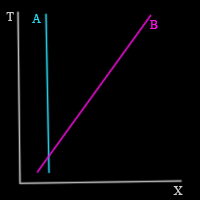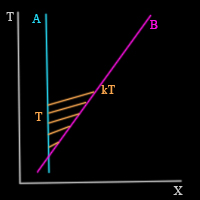Now suppose that A directs a laser beam of light towards B, and leaves it on from the moment they cross paths until some time T, as measured by being A. That light will travel through space, but since the speed of light is not infinite, it will take some time to reach B. And since B is moving away, the final bit of light has to travel further than the first bits of light, and so it will take a little longer to reach B.

We do not yet know how long the light will take to reach B, but we will call the length of time that B is receiving light, k*T where k is an unknown constant.

And so B receives the last bit of light from A at time kT according to B's clock. B wants to let A know that they have received this light signal, and so they immediately send a pulse of light back to A.

This is where the fun begins! The point at which B sends their light back to A is denoted here as p. And because it is a definite point in space and time, both A and B should be able to determine the time and location of this point.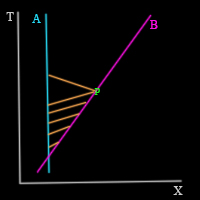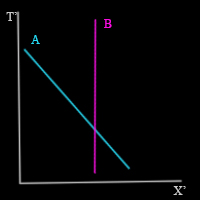As the name suggests, the theory of relativity is based on the assumption that the laws of physics are the same for all beings. It assumes that B can do the same experiment as A and get the same results. And as all experiments before and since the theory of relativity confirm this fact, it is a safe assumption.

And so if we use T' and X ' to  denote the time and space dimensions according to B, we can do the same calculations as we did above, but in B's frame of reference. And that means that if B reflects the light from A for a time T ' = k T, then A will be receiving the light for time k*T '  = k*k*T.

And so now we have all of the times calculated. A emits light for time T, and receives a reply at time k2T. Since the light must travel the same distance and the same length of time in both directions, it is easy for A to calculate that B sent the signal at time tp = ( k2 + 1)T/2 (because this time is halfway between the time when A sent the signal and when they received a reply). And through a similar argument, the position of B at that time must be half the distance that light travels in the time it took to go from A to B and back to A, or xp = ( k2 - 1)c*T/2 where c is the speed of light.

We already know the comparable values in B's frame of reference - the point p occurs at time k*T and because it is part of the path that B follows the spatial position is 0.

We also know that B is travelling at speed v, and so we can immediately see that v = xp/tp = (k2 - 1)c / (k2+1). Using simple algebra, this gives the value of k as k2 = (1 + v/c)/(1 - v/c).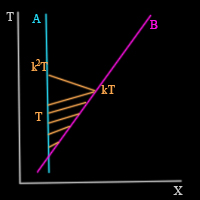This then leads to a most peculiar result. According to B, the event occurs at time T' and according to A the even occurs at time T. And these two values, which pre-relativisitic physics assumes are equal, are actually related by the equation:

T2 = T'2 / ( 1 - (v/c)2)

or in other words, B ages less and sees their clocks running slower than A does. The length of time that passes depends on the motion of the being measuring it. This is the famous time dilation effect, which has been tested in countless experiments and proven to be valid. Using the same equations, it can also be proven that lengths decrease, that energy and effective mass increase, and through those realizations comes the famous equation:

E = m c2

in which mass and energy can be made equivalent.

That is the special theory of relativity in a nutshell. There is no complicated mathematics, or confusing physics, just a few diagrams and a couple of simple assumptions.

As a final parting comment, I will mention that by extending the arguments and equations above to include three spatial dimensions and to two separate points which are located close together (separated in the coordinate system by distances (dx,dy,dz,dt) ), it can be shown that there exists a quantity, written as:

ds2 = dx2 + dy2 + dz2 - c2 dt2

which is the same for every frame of reference. This is refered to as the Minkowski distance (and the equation is called the Minkowski metric) and encodes the entire special theory of relativity. And this is also the first step towards the general theory of relativity - but that is another story.

Make a free website with Yola